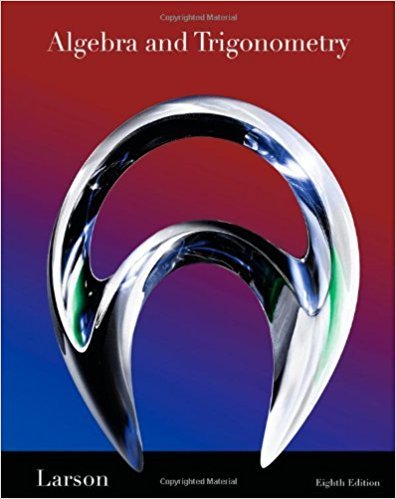×
×

# Solutions for Chapter 4.3: Conics## Full solutions for Algebra and Trigonometry | 8th Edition

ISBN: 9781439048474Solutions for Chapter 4.3: Conics

Solutions for Chapter 4.3
4 5 0 248 Reviews
31
0
##### ISBN: 9781439048474

Since 111 problems in chapter 4.3: Conics have been answered, more than 51198 students have viewed full step-by-step solutions from this chapter. This expansive textbook survival guide covers the following chapters and their solutions. This textbook survival guide was created for the textbook: Algebra and Trigonometry, edition: 8. Chapter 4.3: Conics includes 111 full step-by-step solutions. Algebra and Trigonometry was written by and is associated to the ISBN: 9781439048474.

Key Math Terms and definitions covered in this textbook
• Change of basis matrix M.

The old basis vectors v j are combinations L mij Wi of the new basis vectors. The coordinates of CI VI + ... + cnvn = dl wI + ... + dn Wn are related by d = M c. (For n = 2 set VI = mll WI +m21 W2, V2 = m12WI +m22w2.)

• Cholesky factorization

A = CTC = (L.J]))(L.J]))T for positive definite A.

• Cofactor Cij.

Remove row i and column j; multiply the determinant by (-I)i + j •

• Complex conjugate

z = a - ib for any complex number z = a + ib. Then zz = Iz12.

• Dimension of vector space

dim(V) = number of vectors in any basis for V.

• Four Fundamental Subspaces C (A), N (A), C (AT), N (AT).

Use AT for complex A.

• Gauss-Jordan method.

Invert A by row operations on [A I] to reach [I A-I].

• Gram-Schmidt orthogonalization A = QR.

Independent columns in A, orthonormal columns in Q. Each column q j of Q is a combination of the first j columns of A (and conversely, so R is upper triangular). Convention: diag(R) > o.

• Hermitian matrix A H = AT = A.

Complex analog a j i = aU of a symmetric matrix.

• Identity matrix I (or In).

Diagonal entries = 1, off-diagonal entries = 0.

• Incidence matrix of a directed graph.

The m by n edge-node incidence matrix has a row for each edge (node i to node j), with entries -1 and 1 in columns i and j .

• lA-II = l/lAI and IATI = IAI.

The big formula for det(A) has a sum of n! terms, the cofactor formula uses determinants of size n - 1, volume of box = I det( A) I.

• Multiplicities AM and G M.

The algebraic multiplicity A M of A is the number of times A appears as a root of det(A - AI) = O. The geometric multiplicity GM is the number of independent eigenvectors for A (= dimension of the eigenspace).

• Network.

A directed graph that has constants Cl, ... , Cm associated with the edges.

• Normal matrix.

If N NT = NT N, then N has orthonormal (complex) eigenvectors.

• Row space C (AT) = all combinations of rows of A.

Column vectors by convention.

• Skew-symmetric matrix K.

The transpose is -K, since Kij = -Kji. Eigenvalues are pure imaginary, eigenvectors are orthogonal, eKt is an orthogonal matrix.

• Transpose matrix AT.

Entries AL = Ajj. AT is n by In, AT A is square, symmetric, positive semidefinite. The transposes of AB and A-I are BT AT and (AT)-I.

• Volume of box.

The rows (or the columns) of A generate a box with volume I det(A) I.

• Wavelets Wjk(t).

Stretch and shift the time axis to create Wjk(t) = woo(2j t - k).

×